# Equation - 7th grade (12y) - math problems

#### Number of problems found: 518

• On the farm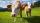On the farm were housed 70 heads of cattle with food supply for 180 days. After 60 days, 10 head of cattle sold, and for the next 30 days they sold 10 more. How many days is sufficient supply of feed for the remaining cattle?
• Nutballs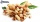The dough for nutballs contains, among other things, two basic raw materials: flour and nuts in a ratio of 2:1. How much flour and how many nuts are needed for 1 kg of dough if "other" is 100g?
• Cost reductionTwo MP3 players whose price was equal to originally have been discounted the first by 20%, the second by 35%. After the price reduction was the difference in their prices 750, - CZK. What was the original price of each of the two players?
• NormThree workers planted 3555 seedlings of tomatoes in one dey. First worked at the standard norm, the second planted 120 seedlings more and the third 135 seedlings more than the first worker. How many seedlings were standard norm?
• Two rooms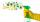Woman cleans the room in 30 minutes a man in 90 minutes. How long will take they clean two rooms?
• Young CyclistsCharlie and Peter will attend the Young Cyclists Meeting today. Peter is still not able to start, so Charlie went first alone. Peter followed him in 20 minutes. How long does he take to reach Charlie? Charlie is traveling at an average speed of 15km/h, Pe
• Percentage - fractionsAbout what percentage we must increase number 1/6 to get number 1/3?
• Two tributaries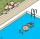Two tributaries of the pool fill it in 10 hours. One of the tributaries would fill 15 hours. How long would fill the first tributary?
• Mysterious number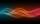The magician thinks the number: "The mysterious number is first divided by minus five, dividing the result by three, multiplying the number by ten, and dividing the resulting number by minus four. This gives result 5. Can you reveal the mysterious number
• Jam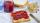Jar with jam weighs 800 grams. Jam weighs three times as much as the empty glass. What is the weight of jam that is in the glass?
• Water containerContainer with water weighs 1.48 kg. When we cast 75% of water container of water weight 0.73 kg. How heavy is an empty container?
• Brothers and sistersLenka has as many brothers as sisters. Her brothers have twice as many sisters as brothers. How many brothers and sisters are in the family?
• Water pool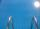Pool with volume 990hl completely filled, if water flows by one tap 8 hours and by second tap 6 hours. First tap give 10hl more than second per hour. How many hl flows in each of them in an hour?
• FactoryIn the factory workers work in three shifts. In the first inning operates half of all employees in the second inning and a third in the third inning 200 employees. How many employees work at the factory?
• Two numbers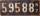Determine the numbers x and y so x + y = 8 is truth and the numbers are in the ratio of 4: 5.
• The ship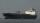The ship went upstream speed of 20 km/h in relation to water. River flows at speed 10 km/h. After half an hour, he stopped and returned downstream to the starting point. How long it took way back when even if downstream speed of ship is 20 km/h in relatio
• Find xSolve: if 2(x-1)=14, then x= (solve an equation with one unknown)
• CandiesThe price for 1 kg of more expensive candies is 125 CZK. The price for 1 kg of cheaper candy is 100 CZK. We mix two different mixtures of candy. A) The first mixture contains 2 kg more expensive and 0.5 kg cheaper candy. Calculate the price for 1 kg of
• Number with onesThe first digit of the number is 1, if we move this digit to the end we get a 3 times higher number, which is the number?
• Truck and car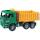At 6h45min the truck started at a speed of 36 km/h. At 7h30m behind it a passenger car. Find the average speed of passenger car if it will catch truck 72 km away from the factory.

Do you have an interesting mathematical word problem that you can't solve it? Submit a math problem, and we can try to solve it.

We will send a solution to your e-mail address. Solved examples are also published here. Please enter the e-mail correctly and check whether you don't have a full mailbox.

Please do not submit problems from current active competitions such as Mathematical Olympiad, correspondence seminars etc...

Do you have a linear equation or system of equations and looking for its solution? Or do you have quadratic equation?